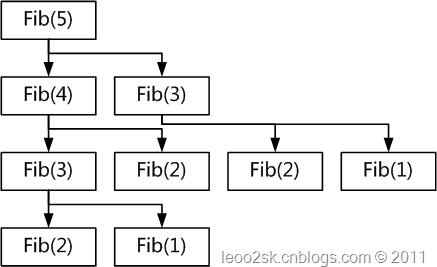## 程序设计中的计算复用(Computational Reuse)

2011-03-03 18:25 by T2噬菌体, ... 阅读, ... 评论, 收藏, 编辑

# 从斐波那契数列说起

public static ulong Fib(ulong n)
{
return (n == 1 || n == 2) ? 1 : Fib(n - 1) + Fib(n - 2);
}public static ulong Fib(ulong n)
{
if (n == 1 || n == 2)
{
return 1;
}
ulong m1 = 1, m2 = 1;
for (ulong i = 3; i <= n; i++)
{
m2 = m1 + m2;
m1 = m2 - m1;
}

return m2;
}

# 计算复用对软件开发的启示

foreach($items as$k => $v){ //...$money = $v->money + getTax(); //... } 当时我问开发这个程序的人这里getTax的返回值和每个item有关系吗，他说税费是一套复杂的算法算出来的，但是其值固定的。那这里可就太浪费了，每次循环都计算一次，如果改为如下： $tax = getTax();
foreach($items as$k => $v){ //...$money = $v->money +$tax;
//...
}

echo count(\$arr);